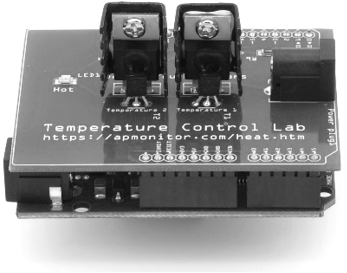## Proportional-only ControlA variation of Proportional Integral Derivative (PID) control is to use only the proportional term as a P-only control. The value of the controller output u(t) is fed into the system as the manipulated variable input.

$$u(t) = u_{bias} + K_c \, \left( SP-PV \right) = u_{bias} + K_c \, e(t)$$

The u_{bias} term is a constant that is typically set to the value of u(t) when the controller is first switched from manual to automatic mode. This gives "bumpless" transfer if the error is zero when the controller is turned on. The one tuning value for a P-only controller is the controller gain, K_c. The value of K_c is a multiplier on the error and a higher value makes the controller more aggressive at responding to any error away from the set point. The set point (SP) is the target value and process variable (PV) is the measured value that may deviate from the desired value. The error from the set point is the difference between the SP and PV and is defined as e(t) = SP - PV.

P-only control is needed for integrating processes (e.g. tank level control with no outlet flow). If used on non-integrating processes there may be persistent offset between the desired set point and process variable with a P-only controller. Integral action is typically used to remove offset (see PI Control).

#### Tuning Correlations

A common tuning correlation for P-only control is the ITAE (Integral of Time-weighted Absolute Error) method. Different tuning correlations are provided for disturbance rejection (also referred to as regulatory control) and set point tracking (also referred to as servo control).

$$K_c = \frac{0.20}{K_p}\left(\frac{\tau_p}{\theta_p}\right)^{1.22} \quad \mathrm{Set\;point\;tracking}$$

$$K_c = \frac{0.50}{K_p}\left(\frac{\tau_p}{\theta_p}\right)^{1.08}\quad \mathrm{Disturbance\;rejection}$$

The parameters K_c, \tau_p, and \theta_p are obtained by fitting dynamic input and output data to a first-order plus dead-time (FOPDT) model.